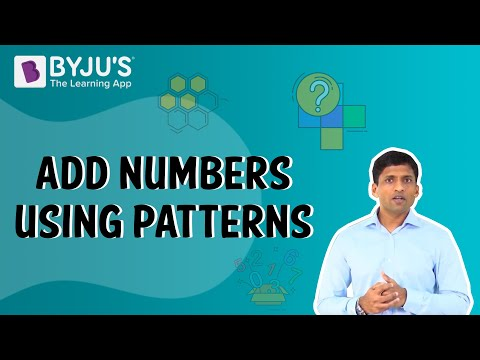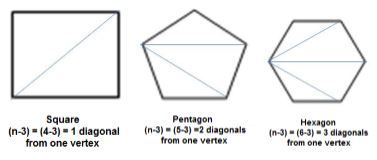# Algebraic Expressions Class 7 Notes: Chapter 12

According to the CBSE Syllabus 2023-24, this chapter has been renumbered as Chapter 10.

## Introduction to Algebraic Expressions

### Constant

Constant is a quantity which has a fixed value.

### Terms of Expression

Parts of an expression which are formed separately first and then added are known as terms. They are added to form expressions.

Example: Terms 4x and 5 are added to form the expression (4x +5).

### Coefficient of a term

The numerical factor of a term is called coefficient of the term.
Example: 10 is the coefficient of the term 10xy in the expression 10xy+4y.

## Algebra as Patterns

#### For more information on Algebra as Patterns, watch the below video.To know more about Algebra as Patterns, visit here.

### Writing Number patterns and rules related to them

• If a natural number is denoted by n, its successor is (n + 1).
Example: Successor of n=10 is n+1 =11.
• If a natural number is denoted by n, 2n is an even number and (2n+1) an odd number.
Example: If n=10, then 2n = 20 is an even number and 2n+1 = 21 is an odd number.To know more about Number patterns, visit here.

### Writing Patterns in Geometry

• Algebraic expressions are used in writing patterns followed by geometrical figures.
Example: Number of diagonals we can draw from one vertex of a polygon of n sides is (n – 3).## Definition of Variables

• Any algebraic expression can have any number of variables and constants.
• ### Variable

• A variable is a quantity that is prone to change with the context of the situation.
• a,x,p,… are used to denote variables.
• To know more about Variables, visit here.

• ### Constant

• It is a quantity which has a fixed value.
• In the expression 5x+4, the variable here is x and the constant is 4.
• The value 5x and 4 are also called terms of expression.
• In the term 5x, 5 is called the coefficient of x. Coefficients are any numerical factor of a term.

### Factors of a term

Factors of a term are quantities which can not be further factorised. A term is a product of its factors.
Example: The term –3xy is a product of the factors –3, x and y.

## Formation of Algebraic Expressions

• Variables and numbers are used to construct terms.
• These terms along with a combination of operators constitute an algebraic expression.
• The algebraic expression has a value that depends on the values of the variables.
• For example, let 6p2−3p+5 be an algebraic expression with variable p
The value of the expression when p=2 is,
6(2)− 3(2) + 5
⇒ 6(4) − 6 + 5 = 23
The value of the expression when p=1 is,
6(1)− 3(1) + 5
⇒ 6 − 3 + 5 = 8

To know more about Algebraic Expressions, visit here.

## Like and Unlike Terms

### Like terms

• Terms having same algebraic factors are like terms.
Example: 8xy and 3xy are like terms.

### Unlike terms

• Terms having different algebraic factors are unlike terms.
Example: 7xy and −3x are unlike terms.

## Monomial, Binomial, Trinomial and Polynomial Terms

### Types of expressions based on the number of terms

Based on the number of terms present, algebraic expressions are classified as:

• Monomial: An expression with only one term.
Example: 7xy, −5m, etc.
• Binomial: An expression which contains two, unlike terms.
Example: 5mn+4, x+y, etc
• Trinomial: An expression which contains three terms.
Example: x+y+5, a+b+ab, etc.

### Polynomials

• An expression with one or more terms.
Example: x+y, 3xy+6+y, etc.

To know more about Polynomial, visit here.

## Addition and Subtraction of Algebraic Equations

• Mathematical operations like addition and subtraction can be applied to algebraic terms.
• For adding or subtracting two or more algebraic expression, like terms of both the expressions are grouped together and unlike terms are retained as it is.
• Sum of two or more like terms is a like term with a numerical coefficient equal to the sum of the numerical coefficients of all like terms.
• Difference between two like terms is a like term with a numerical coefficient equal to the difference between the numerical coefficients of the two like terms.
• For example, 2y + 3x − 2x + 4y
⇒ x(3−2) + y(2+4)
⇒ x+6y
• Summation of algebraic expressions can be done in two ways:
Consider the summation of the algebraic expressions 5a2+7a+2ab and 7a2+9a+11b
• Horizontal method
5a2+7a+2ab+7a2+9a+11b
= (5+7)a2+(7+9)a+2ab+11b
= 12a2+16a+2ab+11b
• Vertical method
5a2+7a+2ab
7a2+9a+11b
¯¯¯¯¯¯¯¯¯¯¯¯¯¯¯¯¯¯¯¯¯¯¯¯¯¯¯¯¯¯¯¯¯¯¯¯¯¯¯¯¯¯¯¯¯¯¯¯¯¯¯¯¯¯
12a2+16a+2ab+11b
¯¯¯¯¯¯¯¯¯¯¯¯¯¯¯¯¯¯¯¯¯¯¯¯¯¯¯¯¯¯¯¯¯¯¯¯¯¯¯¯¯¯¯¯¯¯¯¯¯¯¯¯¯¯

To know more about Addition and Subtraction of Algebraic Equations, visit here.

## Solving an Equation

#### For more information on Solving an Equation, watch the below video.### Use of algebraic expressions in the formula of the perimeter of figures

Algebraic expressions can be used in formulating perimeter of figures.
Example: Let l be the length of one side then the perimeter of:
Equilateral triangle = 3l
Square = 4l
Regular pentagon = 5l

### Use of algebraic expressions in formula of area of figures

Algebraic expressions can be used in formulation area of figures.
Example: Let l be the length and b be the breadth then the area of:
Square = l2
Rectangle = l×b = lb
Triangle =

$$\begin{array}{l}\frac{b\times h}{2}\end{array}$$
, where b and h are base and height, respectively.

## Frequently Asked Questions on CBSE Class 7 Maths Notes Chapter 12 Algebraic Expressions

Q1

### What is a constant?

Constant is a quantity which has a fixed value.

Q2

### What are like and unlike terms?

In algebra, like terms are terms that have the same variables and powers. Unlike terms are two or more terms that are not like terms, i.e. they do not have the same variables or powers.

Q3

### What are the types of polynomials?

1. Monomial 2. Binomial 3. Trinomial